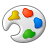# SRAM Forum DiscussionsAnonymous
Not applicable

# IO Power Calculation in SRAMs

The operating current ICC mentioned in the datasheet includes only the core power, it does not include the IO power. You can compute the IO power as shown below.

Assuming capacitive load only, IO power can be estimated to be ((α)* f *N * CL* V * V)
where
α is called the activity factor ; if on an average half the IO's switch per clock cycle, then α is 0.5.
f is the switching frequency or clock frequency
N is the number of IO's
V is the Voltage swing of the IO.

For example: IO power for (CYD18S36V18) = (10MHz * 36 * 20pF * 3.3^2)/2 = 39.2mW.

3 RepliesAnonymous
Not applicable

# Re: IO Power Calculation in SRAMs

They have created web tools for power calculations of Async and Sync SRAMs.Employee
Employee# Re: IO Power Calculation in SRAMs

Hello Abhu,